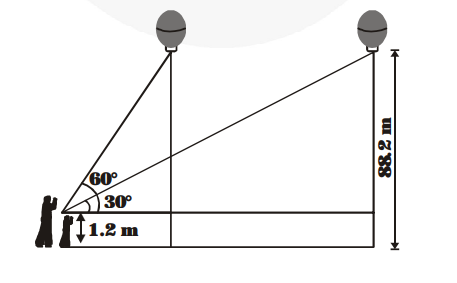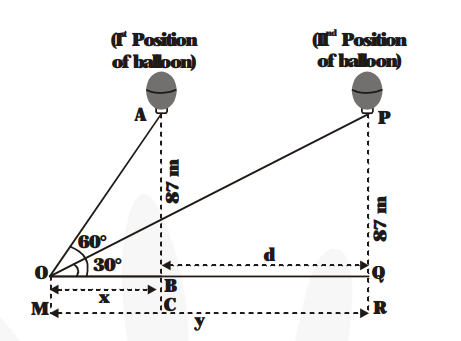# A 1.2 m tall girl spots a balloon moving with the wind in a horizontal line at a height of 88.2 m from the ground.Question:

A 1.2 m tall girl spots a balloon moving with the wind in a horizontal line at a height of 88.2 m from the ground. The angle of elevation of the balloon from the eyes of the girl at any instant is 60°. After some time, the angle of elevation reduces to 30°. Find the distance travelled by the balloon during the interval.Solution:

From figure, we have $\angle \mathrm{POQ}=30^{\circ}$ is the angle of elevation for the first position of the balloon. Let

$\mathrm{OQ}=\mathrm{y} \mathrm{m}$We are given that

AC = 88.2 m., AB = 88.2 – 1.2 = 87 m

For the second position of the balloon, we have

$\angle \mathrm{POQ}=30^{\circ} .$ Let $\mathrm{OB}=\mathrm{x} \mathrm{m}$

We have to find $d=B Q=(y-x)$

$\frac{\mathbf{A B}}{\mathbf{D B}}=\tan \mathbf{6 0}^{\circ}$ and $\frac{\mathbf{P Q}}{\mathbf{D Q}}=\tan 30^{\circ}$

$\Rightarrow \frac{87}{x}=\sqrt{3}$ and $\frac{87}{y}=\frac{1}{\sqrt{3}}$

$\Rightarrow \frac{87}{x}=\sqrt{3}$ and $\frac{87}{y}=\frac{1}{\sqrt{3}}$

$\Rightarrow x=\frac{87}{\sqrt{3}} m$ and $y=87 \sqrt{3} m$

Then $d=y-x=\left\{\mathbf{8 7} \sqrt{\mathbf{3}}-\frac{\mathbf{8 7}}{\sqrt{\mathbf{3}}}\right\} \mathbf{m}$

$=87 \times\left\{\sqrt{3}-\frac{1}{\sqrt{3}}\right\} \mathbf{m}$

$=87 \times \frac{\boldsymbol{2}}{\sqrt{\mathbf{3}}} \mathrm{m}=87 \times \frac{\boldsymbol{2}}{\boldsymbol{3}} \times \sqrt{\mathbf{3}} \mathrm{m}$

$=\frac{174}{3} \sqrt{3} \mathrm{~m}=58 \sqrt{3} \mathrm{~m}$